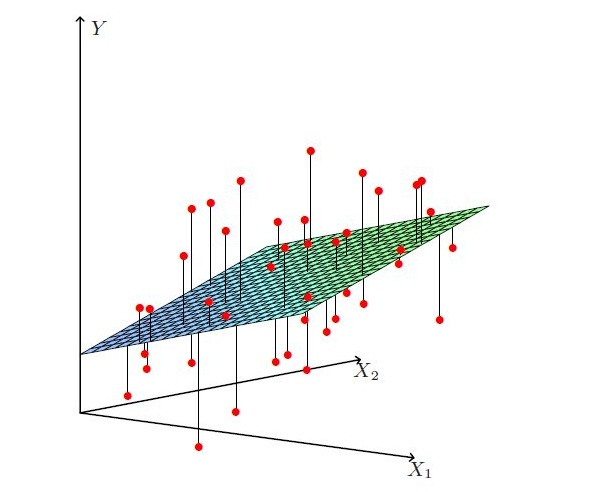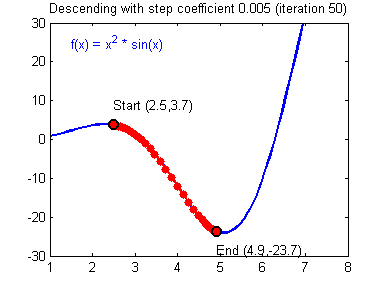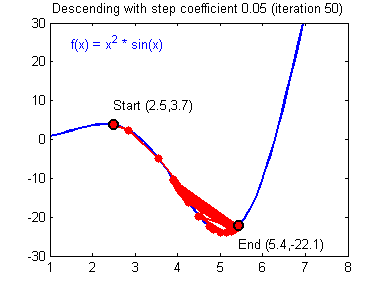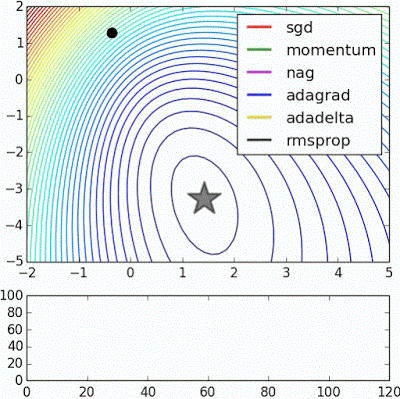# 线性回归的求解：矩阵方程和梯度下降、数学推导及NumPy实现

## Normal Equation

### 一元线性回归

\begin{aligned} \frac{\partial}{\partial a}L(a, b) &= \frac{2}{m}\sum_{i=1}^mx^{(i)}(ax^{(i)} + b -y^{(i)}) \\ &=\frac{2a}{m}\sum_{i=1}^m{x^{(i)}}^2 + \frac{2b}{m}\sum_{i=1}^mx^{(i)}-\frac{2}{m}\sum_{i=1}^m{x^{(i)}y^{(i)}} \\ &= \frac{2}{m}(a\sum_{i=1}^m{x^{(i)}}^2 + b\sum_{i=1}^mx^{(i)} - \sum_{i=1}^m{x^{(i)}y^{(i)}}) \\ &= \frac{2}{m}(a\sum_{i}^m{x^{(i)}}^2-\sum_{i=1}^m(y^{(i)}-b)x^{(i)}) \end{aligned} \tag{2}

\begin{aligned} \frac{\partial}{\partial b}L(a, b) &= \frac{2}{m}\sum_{i=1}^max^{(i)}+b-y^{(i)} \\ &= \frac{2a}{m}\sum_{i=1}^m{x^{(i)}} + 2b - \frac{2}{m}\sum_{i=1}^m{y^{(i)}} \\ &= \frac{2}{m}(\sum_{i=1}^max^{(i)} + mb - \sum_{i=1}^my^{(i)}) \\ &= \frac{2}{m}(mb - \sum_{i=1}^m(y^{(i)} -ax^{(i)})) \end{aligned} \tag{3}

$\frac{\partial}{\partial a}L(a, b) = 0 \Rightarrow \frac{2}{m}(a\sum_{i=1}^m{x^{(i)}}^2-\sum_{i=1}^m(y^{(i)}-b)x^{(i)}) = 0 \tag{4}$

$\frac{\partial}{\partial b}L(a, b) = 0 \Rightarrow \frac{2}{m}(mb - \sum_{i=1}^m(y^{(i)} -ax^{(i)})) = 0 \tag{5}$

$a^*=\frac{\sum_{i=1}^my^{(i)}(x^{(i)}-\bar{x})}{\sum_{i=1}^m{x^{(i)}}^2-\frac{1}{m}(\sum_{i=1}^mx^{(i)})^2} \tag{6}$ $b^*=\frac{1}{m}\sum_{i=1}^m(y^{(i)}-ax^{(i)})$

### 多元线性回归

$f(\boldsymbol{x}^{(i)}) = \boldsymbol{w}^T \boldsymbol{x}^{(i)} \tag{7}$多元线性回归一般寻找最优超平面

$L(\boldsymbol{w}) = (\boldsymbol{X}\boldsymbol{w}-\boldsymbol{y})^\top (\boldsymbol{X}\boldsymbol{w}-\boldsymbol{y}) = ||\boldsymbol{X}\boldsymbol{w}-\boldsymbol{y}||_2^2 \tag{10}$ 多元线性回归的损失函数仍然使用“预测值-真实值”的平方来计算，公式10为整个模型损失函数的向量表示。这里出现了一个竖线组成的部分，它被称作L2范数的平方。范数通常针对向量，也是一个机器学习领域经常用到的数学符号，公式11展示了一个向量 $\boldsymbol{x}$ 的L2范数的平方以及其导数。 $\\ ||\boldsymbol{x}||_2^2 = ((\sum_{i=1}^N{x^{(i)}}^2)^{\frac{1}{2}})^2 \qquad \qquad \qquad \qquad \nabla||\boldsymbol{x}||_2^2=2\boldsymbol{x} \tag{11}\\$

$\frac{\partial}{\partial \boldsymbol{w}}L(\boldsymbol{w}) = 2\boldsymbol{X}^\top (\boldsymbol{X}\boldsymbol{w} - \boldsymbol{y}) = 0 \tag{12}$

$\boldsymbol{X}^\top\boldsymbol{X}\boldsymbol{w} = \boldsymbol{X}^\top \boldsymbol{y} \Rightarrow (\boldsymbol{X}^\top\boldsymbol{X})^{-1} \boldsymbol{X}^\top\boldsymbol{X}\boldsymbol{w}=(\boldsymbol{X}^\top\boldsymbol{X})^{-1} \boldsymbol{X}^\top \boldsymbol{y} \tag{13}$

$\boldsymbol{w}^* = (\boldsymbol{X}^\top\boldsymbol{X})^{-1}\boldsymbol{X}^\top \boldsymbol{y} \tag{14}$

## 梯度下降法损失函数沿梯度下降的过程收敛速度过慢的情形

$w_j := w_j - \alpha (f(\boldsymbol{x}^{(i)})-y^{(i)}) x_{j}^{(i)}$

• 更新的大小与$(f(\boldsymbol{x}^{(i)})-y^{(i)})$成比例。
• 当我们遇到训练样本的预测值$f(\boldsymbol{x}^{(i)})$$y^{(i)}$ 的真实值非常接近的情况下，就会发现基本没必要再对参数进行修改了；与此相反的情况是，如果我们的预测值 $f(\boldsymbol{x}^{(i)})$$y^{(i)}$真实值有很大的误差（比如距离特别远），那就需要对参数进行更大地调整。这也与前面所展示的梯度下降动态图中相吻合。

### 批量梯度下降法

\begin{aligned} \frac \partial {\partial w_j}L(w) & = \frac \partial {\partial w_j} \frac 12\lbrace (f(\boldsymbol{x}^{(1)}) - y^{(1)})^2 + \cdots + (f(\boldsymbol{x}^{(m)}) - y^{(m)})^2 \rbrace\\ & = 2 \cdot\frac 12(f(\boldsymbol{x}^{(1)})-y^{(1)})\cdot \frac \partial {\partial w_j} (f(\boldsymbol{x}^{(1)})-y^{(1)}) + \cdots\\ & = (f(\boldsymbol{x}^{(1)})-y^{(1)})\cdot \frac \partial {\partial w_j}(\sum^n_{j=0} w_jx_{j}^{(1)}-y^{(1)}) + \cdots \\ & = (f(\boldsymbol{x}^{(1)})-y^{(1)}) x_{j}^{(1)} + \cdots \\ & = \sum_{i=1}^m(f(\boldsymbol{x}^{(i)}) - y^{(i)})x_{j}^{(i)} \end{aligned}

### 随机梯度下降法不同梯度下降法的收敛速度示意图

### 梯度下降法的NumPy实现

import numpy as np

class LinearRegression:

def __init__(self):
# the weight vector
self.W = None

def train(self, X, y, method='bgd', learning_rate=1e-2, num_iters=100, verbose=False):
"""

Parameters
----------
X: training data, shape (num_of_samples x num_of_features), num_of_samples rows of training sample, each training sample has num_of_features-dimension features.
y: target, shape (num_of_samples, 1).
method: (string) 'bgd' for Batch Gradient Descent or 'sgd' for Stochastic Gradient Descent
learning_rate: (float) learning rate or alpha
num_iters: (integer) number of steps to iterate for optimization
verbose: (boolean) if True, print out the progress

Returns
-------
losses_history: (list) of losses at each training iteration
"""
num_of_samples, num_of_features = X.shape

if self.W is None:
# initilize weights with values
# shape (num_of_features, 1)
self.W = np.random.randn(num_of_features, 1) * 0.001
losses_history = []

for i in range(num_iters):

if method == 'sgd':
# randomly choose a sample
idx = np.random.choice(num_of_samples)
else:
losses_history.append(loss)

# Update weights using matrix computing (vectorized)

if verbose and i % (num_iters / 10) == 0:
print('iteration %d / %d : loss %f' %(i, num_iters, loss))
return losses_history

def predict(self, X):
"""
Predict value of y using trained weights

Parameters
----------
X: predict data, shape (num_of_samples x num_of_features), each row is a sample with num_of_features-dimension features.

Returns
-------
pred_ys: predicted data, shape (num_of_samples, 1)
"""
pred_ys = X.dot(self.W)
return pred_ys

"""

Parameters
----------
The same as self.train function

Returns
-------
tuple of two items (loss, gradient)
loss: (float)
gradient: (array) with respect to self.W
"""
if vectorized:
else:

"""
Compute the loss and gradients with weights, vectorized version
"""
# vectorized implementation
num_of_samples = X.shape
# (num_of_samples, num_of_features) * (num_of_features, 1)
f_mat = X.dot(W)

# (num_of_samples, 1) - (num_of_samples, 1)
diff = f_mat - y
loss = 1.0 / 2 * np.sum(diff * diff)

# {(num_of_samples, 1).T dot (num_of_samples, num_of_features)}.T

"""
Compute the loss and gradients with weights, for loop version
"""

# num_of_samples rows of training data
num_of_samples = X.shape

# num_of_samples columns of features
num_of_features = X.shape

loss = 0

# shape (num_of_features, 1) same with W

for i in range(num_of_samples):
X_i = X[i, :] # i-th sample from training data
f = 0
for j in range(num_of_features):
f += X_i[j] * W[j, 0]
diff = f - y[i, 0]
loss += np.power(diff, 2)
for j in range(num_of_features):
gradient[j, 0] += diff * X_i[j]

loss = 1.0 / 2 * loss